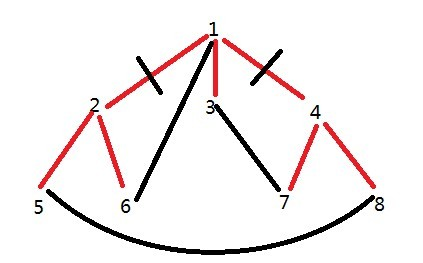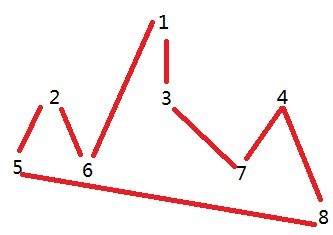# Tree2cycle

Time Limit: 15000/8000 MS (Java/Others)    Memory Limit: 102400/102400 K (Java/Others)
Total Submission(s): 382    Accepted Submission(s): 74

Problem Description
A tree with N nodes and N-1 edges is given. To connect or disconnect one edge, we need 1 unit of cost respectively. The nodes are labeled from 1 to N. Your job is to transform the tree to a cycle(without superfluous edges) using minimal cost.

A cycle of n nodes is defined as follows: (1)a graph with n nodes and n edges (2)the degree of every node is 2 (3) each node can reach every other node with these N edges.

Input
The first line contains the number of test cases T( T<=10 ). Following lines are the scenarios of each test case.
In the first line of each test case, there is a single integer N( 3<=N<=1000000 ) - the number of nodes in the tree. The following N-1 lines describe the N-1 edges of the tree. Each line has a pair of integer U, V ( 1<=U,V<=N ), describing a bidirectional edge (U, V).

Output
For each test case, please output one integer representing minimal cost to transform the tree to a cycle.

Sample Input
1 4 1 2 2 3 2 4

Sample Output
3
Hint
In the sample above, you can disconnect (2,4) and then connect (1, 4) and (3, 4), and the total cost is 3.

Source

Recommend
liuyiding#pragma comment(linker,"/STACK:102400000,102400000")
#include <cstdio>
#include <cstring>
#include <algorithm>
using namespace std;
const int V = 1000000 + 50;
int pnt[V * 2], nxt[V * 2], head[V * 2], e;
int T, n, ans;
bool vis[V];
inline void addedge(int u, int v) {
pnt[e] = v;
nxt[e] = head[u];
head[u] = e++;
}
bool dfs(int u) { //返回是否还是还是u的儿子。
int sum = 0;
vis[u] = true;
for(int i = head[u]; i != -1; i = nxt[i])
if(!vis[pnt[i]])
sum += dfs(pnt[i]);
if(sum >= 2) {
if(u == 1) //如果是根节点
ans += 2 * (sum - 2);
else
ans += 2 * (sum - 1);
return false;
}
else
return true;
}
int main() {
int i, j, k;
scanf("%d", &T);
while(T--) {
scanf("%d", &n);
e = ans = 0;
memset(head, -1, sizeof(head));
memset(vis, false, sizeof(vis));
for(i = 0; i < n - 1; ++i) {
int a, b;
scanf("%d%d", &a, &b);
addedge(a, b);
addedge(b, a);
}
dfs(1);
printf("%d\n", ans + 1);
}
}